# Topic 11: Electromagnetic induction (HL)

See the guide for this topic.

## 11.1 – Electromagnetic induction

• ### Electromotive force (emf)

• When a conducting wire moves through a magnetic field, a potential difference is created along the wire. This phenomenon is called electromagnetic induction.• When the movement of the wire is perpendicular to the magnetic field, the emf (ε) induced is given by ε = Bvl where B is the magnetic field, v is the velocity of the wire, and l is the length of the wire.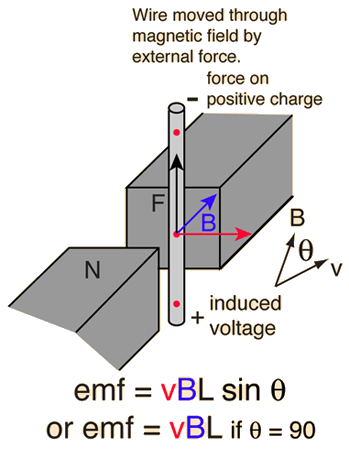• ### Magnetic flux and magnetic flux linkage

• The magnetic flux through a surface measures the component of the magnetic field passing through the surface and is proportional to the number of magnetic field lines which intersect the surface.
• The magnetic flux (φ) through a surface is given by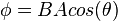where B is the magnetic field passing through the surface, A is the area of the surface, and θ is the angle between the magnetic field and the normal to the surface.• The magnetic flux linkage of a coil measures the component of the magnetic field passing through the coil.
• For a coil with N turns, the total flux linkage is given bywhere BA = φ = magnetic flux

• ### Faraday’s law of induction

• Faraday’s law states that the magnitude of the induced emf is proportional to the rate of change of the flux linkage by• Faraday’s law allows us to determine the induced emf by the change of magnetic flux over time.

• ### Lenz’s law

• Lenz’s law states that the induced emf acts in the direction such that the current induced opposes the change which caused it.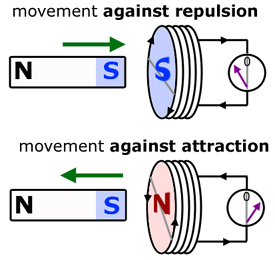## 11.2 – Power generation and transmission

• ### Alternating current (ac) generators

• The operation of a basic alternating current (ac) generator is demonstrated in the following diagram.1. A coil of wire is driven to rotate by an external force.
2. As the coil rotates, the magnetic flux linkage passing through the coil changes.
3. By Faraday’s law, this induces an emf and causes current to flow within the coil.
• If the rotation is at constant speed, the induced emf is sinusoidal (recurring oscillation).
• Increasing the speed of rotation increases both the frequency and the magnitude of the induced emf.• ### Average power and root mean square (rms) values of current and voltage

• The average power produced (or dissipated) from an alternating current cannot be computed directly using the peak values of voltage or current.
• The root mean square (rms) value of an alternating current or voltage is the value that could be substituted into the electrical power formula P=IV to calculate the average power dissipated where I and V are rms values.where V0 is the peak value of the alternating voltage.where I0 is the peak value of the alternating current.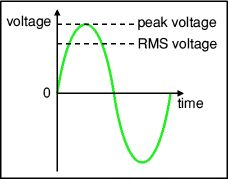• The quoted values for alternating voltage and current refer to their rms values rather than their peak values. For an example, an ac socket in Europe is rated at 220V rms.

• ### Transformers

• A transformer is a device which can be used to transfer electrical energy from one ac circuit to another at a different voltage.
• Transformers which increase the output voltage are called step up transformers while those which decrease the output voltage are called step down transformers.
• A transformer is essentially two coil coils of wire coupled together as shown below.1. Alternating input voltage causes constant change in the magnetic field around the primary coil.
2. This causes a constant change in the magnetic flux linkage in the secondary coil.
3. By Faraday’s law, an emf is induced in the secondary coil.
• The ratio between the induced input voltage (rms) and the output voltage (rms) is equal to the ratio between the number of turns in the corresponding coil.where Vp is the voltage in the primary coil, Vs is the voltage in the secondary coil, np is the number of turns on the primary coil, and ns is the number of turns on the secondary coil.

• An ideal transformer operates at 100% efficiency. In other words, its power input is equal to power output.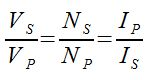since P=IV

• ### Diode bridges

A diode bridge is an arrangement of four (or more) diodes in a bridge circuit configuration where a diode is a two-terminal electronic component that conducts current to flow in one direction.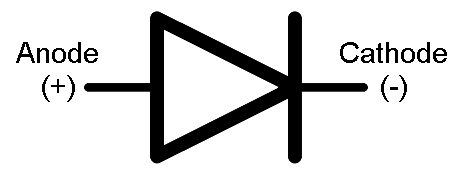• ### Half-wave and full-wave rectification

Diodes are also known as rectifiers. They can be used to change alternating current (ac) to direct current (dc) through a process called rectification.

Half-wave rectification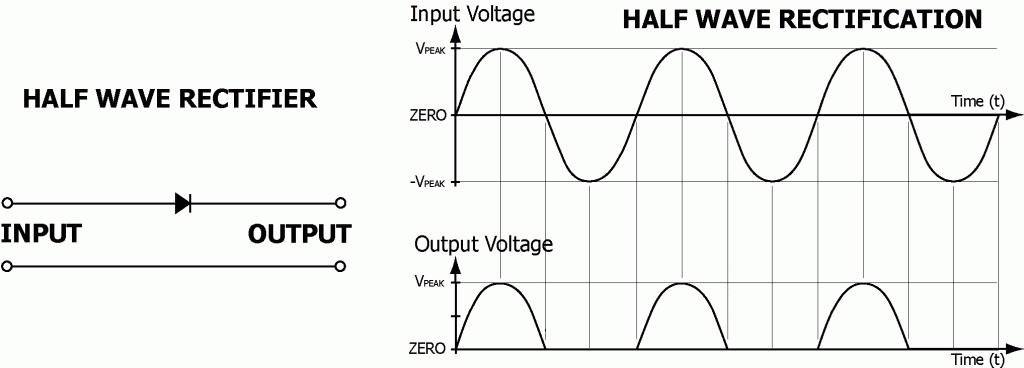The final dc waveform on the screen is the positive half only of the original ac waveform. In half-wave rectification, the negative part of the current is prevented from passing.

Full-wave rectification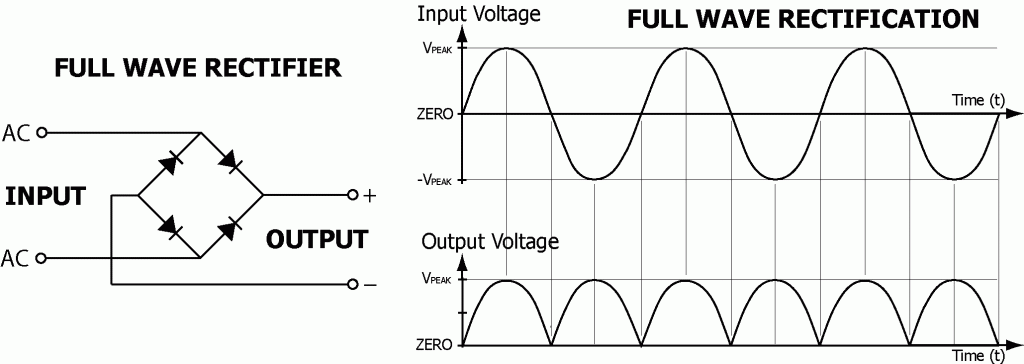The four diodes connected as shown in the diagram above form a full-wave rectifier. Through this arrangement, the positive half of each cycle is allowed to pass through while the negative half of each cycle is reversed. This diode configuration is commonly known as a diode bridge.

## 11.3 – Capacitance

• ### Capacitance

Capacitance (C) is the ability to store change given in the unit farad (F) and can be expressed aswhere C is capacitance, Q is charge, and V is voltage.A capacitor is made up of two metallic plates with a dielectric material in between the plates.When a voltage is applied over the two plates, an electric field is created with positive charge collecting in one plate and negative charge on the other.

This is what physicists mean when they say “a capacitor works by storing energy electrostatically in an electric field”.

The capacitance of a capacitor can be correlated to the area of the plates (A) and the distance of separation between the plates (d) by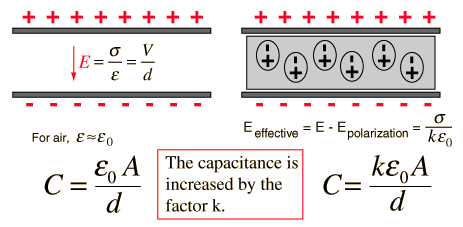### FYI

The potential energy stored in a capacitor is given bywhere E is the potential energy, Q is charge, V is voltage, and C is capacitance.

• ### Dielectric materials

• Dielectric materials are insulators, such as glass, plastics, distilled water, dry air, or even a vacuum.
• Dielectric materials keep the conducting plates in contact, allowing for smaller plate separation (d), and higher capacitance (C).

• ### Capacitors in series and parallel• ### Resistor-capacitor (RC) series circuits

An RC circuit is one where you have a capacitor and resistor in the same circuit.The charging and discharging of an RC circuit works like this:

1. Assuming that C is fully discharged and that the switch is open. When the switch is closed in position 1, the battery is connected across the capacitor. Current flows and the potential difference across the capacitor begins to rise but as more and more charge build up on the capacitor plates, the current and the rate of rise of potential difference both fall until no further current flows and the potential difference across the capacitor equals that of the supply voltage (V0). The capacitor is now fully charged.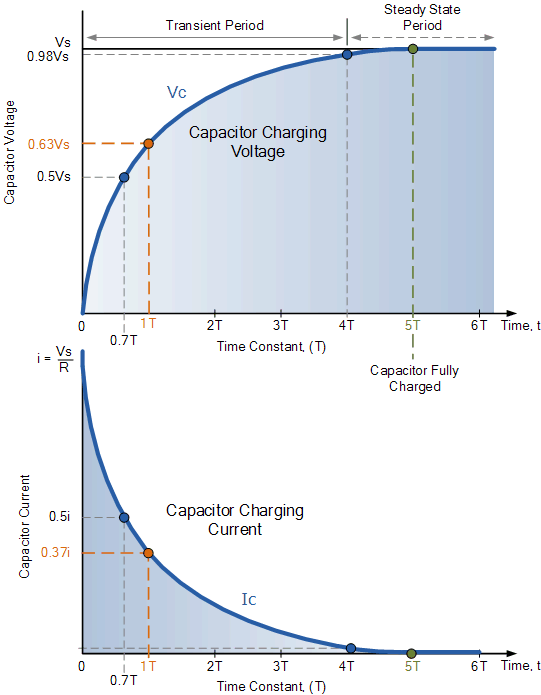1. When the switch is then closed at position 2, a large current flows and the potential difference across the capacitor drops. As charge flows from one plate to the other through the resistor, the charge is neutralized. The current and the rate of decreasing potential difference both fall. When the charge on the plates reach zero, the initial condition, the current and potential difference are also zero. The capacitor is now fully discharged.• ### Time constant

The RC time constant specifies the time required to charge the capacitor, through a resistor, by approximately 63.2% or to discharge the capacitor, through a resistor, by approximately 36.8% and is given bywhere τ is the RC time constant in seconds, R is the circuit resistance in ohms, and C is the circuit capacitance in farads.